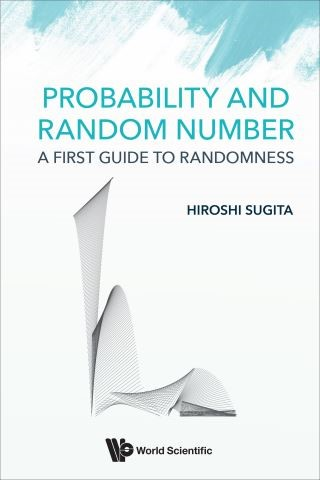## Probability and Random Number• Description
• Author
• Info
• Reviews

### Description

This is a book of elementary probability theory that includes a chapter on algorithmic randomness. It rigorously presents definitions and theorems in computation theory, and explains the meanings of the theorems by comparing them with mechanisms of the computer, which is very effective in the current computer age.

Random number topics have not been treated by any books on probability theory, only some books on computation theory. However, the notion of random number is necessary for understanding the essential relation between probability and randomness. The field of probability has changed very much, thus this book will make and leave a big impact even to expert probabilists.

Readers from applied sciences will benefit from this book because it presents a very proper foundation of the Monte Carlo method with practical solutions, keeping the technical level no higher than 1st year university calculus.

Contents:
• Mathematics of Coin Tossing
• Mathematical Model
• Random Number
• Limit Theorem
• Monte Carlo Method
• Infinite coin Tosses
• Random Number:
• Recursive Function
• Kolmogorov Complexity and Random Number
• Limit Theorem:
• Bernoulli's Theorem
• Law of Large Numbers
• De Moivre–Laplace's Theorem
• Central Limit Theorem
• Mathematical Statistics
• Monte Carlo Method:
• Monte Carlo Method as Gambling
• Pseudorandom Generator
• Monte Carlo Integration
• From the Viewpoint of Mathematical Statistics
• Appendices:
• Symbols and Terms
• Binary Numeral System
• Limit of Sequence and Function
• Limits of Exponential Function and Logarithm
• C Language Program

Readership: First year university students to professionals.
Keywords:Probability;Probability Theory;Randomness;Random Number;Pseudorandom Number;Monte Carlo Method;Monte Carlo IntegrationReview:Key Features:
• This is the first book that presents both probability theory and algorithmic randomness for from 1st year university students to experts. It is technically easy but worth reading for experts as well
• This book presents basic limit theorems with proofs that are not seen in usual probability textbooks; for readers should learn that a good solution is not always unique
• This book rigorously treats the Monte Carlo method. In particular, it presents the random Weyl sampling, which produces pseudorandom numbers for the Monte Carlo integration that act complete substitutes for random numbers